Practice the AP 7th Class Maths Bits with Answers Chapter 14 Understanding 3D and 2D Shapes on a regular basis so that you can attempt exams with utmost confidence.

## AP State Syllabus 7th Class Maths Bits 14th Lesson Understanding 3D and 2D Shapes with Answers

Question 1.
The net of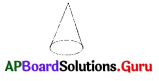is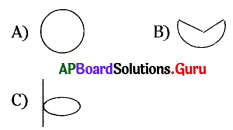D) none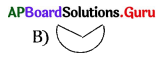Question 2.
Identify the net shape of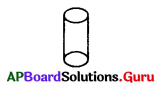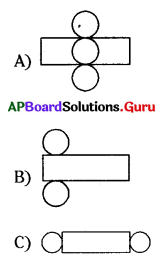D) none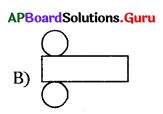Question 3.
Identify the net shape of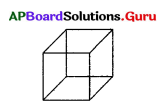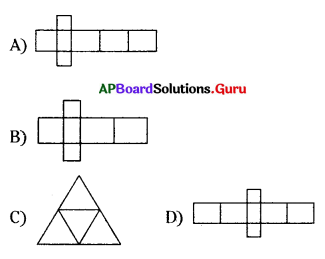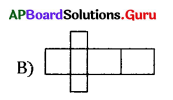Question 4.
What is the vertical shape of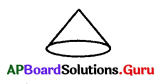?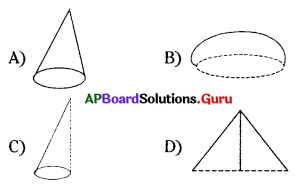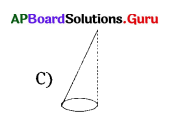Question 5.
The Horizontal cut of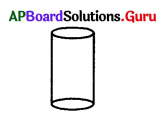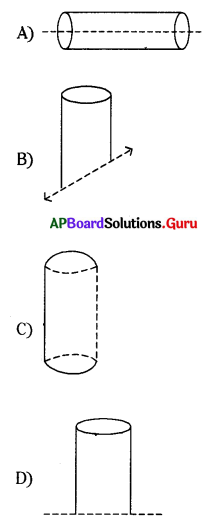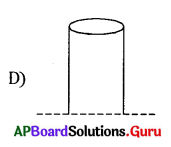Question 6.
When spherical shape seen under the lamp of an overhead projector it seems to
be a shape of ……………..
A) square
B) circle
C) rectangle
D) triangle
B) circle

Question 7.
From the above sum cube is ……………….
A) circle
B) square
C) rectangle
D) triangle
B) square

Question 8.
Textbook is in the shape of
A) rectangle
B) square
C) circle
D) triangle
A) rectangle

Question 9.
A cube has ………………… edges.
A) 4
B) 8
C) 12
D) 6
C) 12Question 10.
A cuboid has ……………….. faces.
A) 4
B) 6
C) 8
D) 12
B) 6

Question 11.
The skelton out line of a solid on 2-D surface is called
A) opaque sketch
B) isometric sketch
C) net
D) none
C) net

Question 12.
The opposite faces of a dice always have a total of …………….. on them.
A) 9
B) 8
C) 7
D) 6
C) 7

Question 13.
Which of the following is a 3-D shape?
A) Triangle
B) Rectangle
C) Cone
D) Square
C) Cone

Question 14.
Which of the following is not a 3-D shape?
A) Parallelogram
B) Cylinder
C) Sphere
D) Cone
A) Parallelogram

Question 15.
Cricket ball is an example of ………………. shape.
A) sphere
B) cone
C) cylinder
D) none
A) sphere

Question 16.
Example for a cube is ……………..
A) pen
B) dice
C) ice cream-cone
D) none
B) dice

Question 17.
Example for a cylindrical shape is ……………….
A) Candle
B) Brick
D) Tin
A) Candle

Question 18.
Number of faces of a cube are ………………
A) 6
B) 7
C) 8
D) 5
A) 6

Question 19.
Number of edges of a pyramid are ………………..
A) 12
B) 10
C) 8
D) 9
C) 8

Question 20.
Number of vertices of a cuboid are ……………..
A) 6
B) 4
C) 5
D) 8
D) 8

II. Matching:

1. Match the following.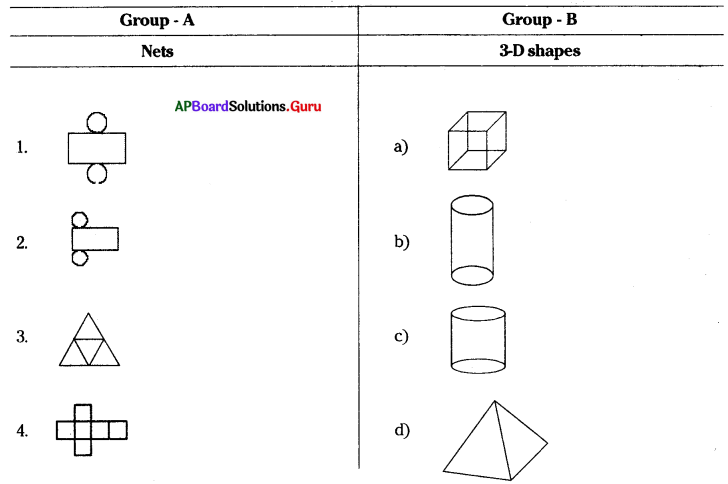1) – b
2) – c
3) – d
4) – a

2. Match the following.

 Group – A Group – B Object Its shadows 1. A football a) Triangular 2. A triangular prism b) Rectangular 3. A dice c) Pyramidical 4. A notebook d) Cuboid 5. A cylindrical pipe e) Square f) Circular